# W-M5P

Member Posts: 19Contributor I
in Help
Hello everyone,

I am using the W-M5P from the weka extension to get a model tree and be able to extract rules. However, I always get only one rule (shown in the screenshot) and also I don't get the graph of the tree at all unlike with the rapidminer regression tree or random forest.

It would be great if anyone could help here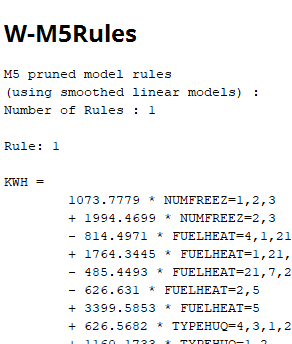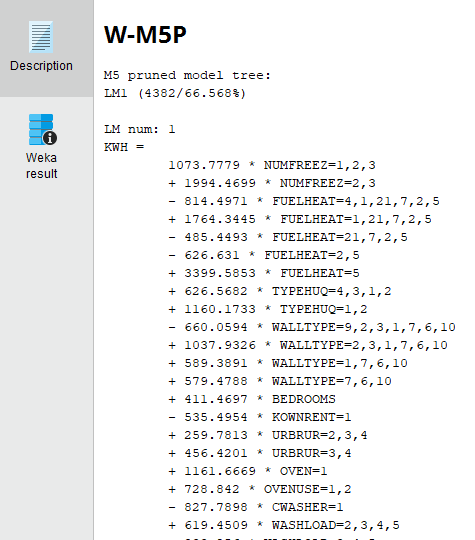Tagged:

• Moderator, Member Posts: 964Unicorn
Hello @islem_h

I tried with the polynomial dataset in RapidMiner Samples. I can get multiple rules using W-M5P or W-M5Rules operators from weka extension. I can also generate the tree (Screenshots below). It mainly depends on your data. If you can provide your XML process and dataset we can try to replicate and see what is happening. Sample code used attached in this as well.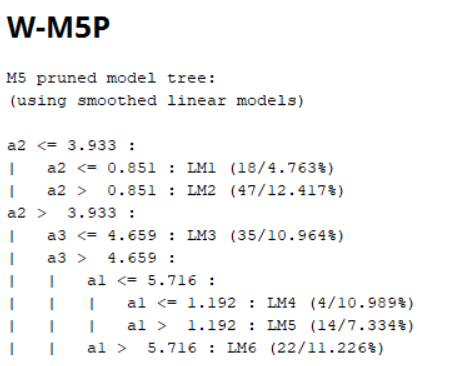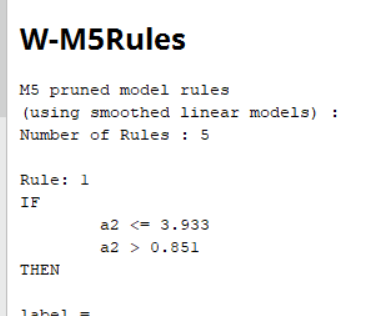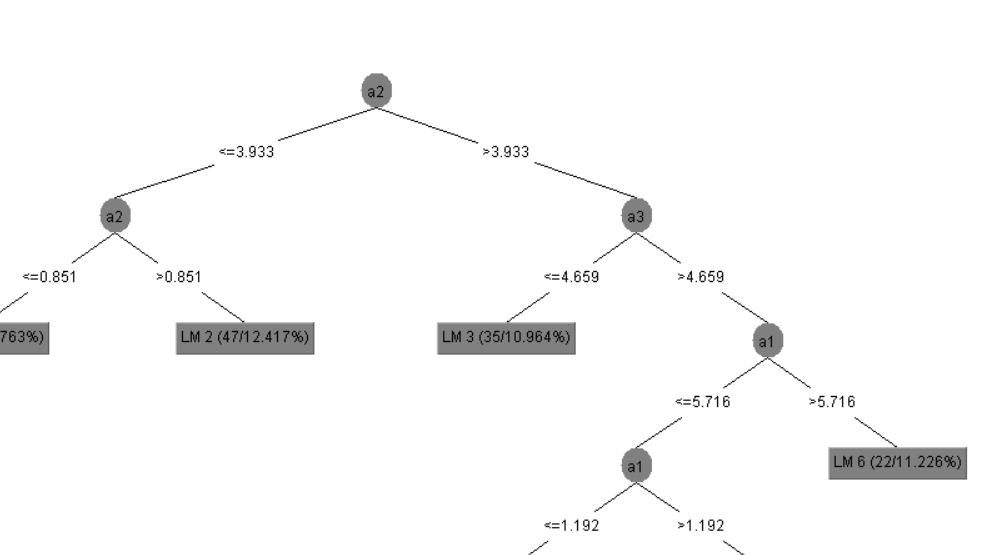```<?xml version="1.0" encoding="UTF-8"?><process version="9.2.001">
<context>
<input/>
<output/>
<macros/>
</context>
<operator activated="true" class="process" compatibility="9.2.001" expanded="true" name="Process">
<parameter key="logverbosity" value="init"/>
<parameter key="random_seed" value="2001"/>
<parameter key="send_mail" value="never"/>
<parameter key="process_duration_for_mail" value="30"/>
<parameter key="encoding" value="SYSTEM"/>
<process expanded="true">
<operator activated="true" class="retrieve" compatibility="9.2.001" expanded="true" height="68" name="Retrieve Polynomial" width="90" x="45" y="85">
<parameter key="repository_entry" value="//Samples/data/Polynomial"/>
</operator>
<operator activated="true" class="split_data" compatibility="9.2.001" expanded="true" height="103" name="Split Data" width="90" x="179" y="85">
<enumeration key="partitions">
<parameter key="ratio" value="0.7"/>
<parameter key="ratio" value="0.3"/>
</enumeration>
<parameter key="sampling_type" value="automatic"/>
<parameter key="use_local_random_seed" value="false"/>
<parameter key="local_random_seed" value="1992"/>
</operator>
<operator activated="false" class="weka:W-M5P" compatibility="7.3.000" expanded="true" height="82" name="W-M5P" width="90" x="380" y="442">
<parameter key="N" value="false"/>
<parameter key="U" value="false"/>
<parameter key="R" value="false"/>
<parameter key="M" value="4.0"/>
<parameter key="L" value="false"/>
</operator>
<operator activated="true" class="weka:W-M5P" compatibility="7.3.000" expanded="true" height="82" name="W-M5P (2)" width="90" x="313" y="34">
<parameter key="N" value="false"/>
<parameter key="U" value="false"/>
<parameter key="R" value="false"/>
<parameter key="M" value="4.0"/>
<parameter key="L" value="false"/>
</operator>
<operator activated="true" class="apply_model" compatibility="9.2.001" expanded="true" height="82" name="Apply Model" width="90" x="447" y="136">
<list key="application_parameters"/>
<parameter key="create_view" value="false"/>
</operator>
<operator activated="true" class="performance_regression" compatibility="9.2.001" expanded="true" height="82" name="Performance" width="90" x="514" y="34">
<parameter key="main_criterion" value="first"/>
<parameter key="root_mean_squared_error" value="true"/>
<parameter key="absolute_error" value="false"/>
<parameter key="relative_error" value="false"/>
<parameter key="relative_error_lenient" value="false"/>
<parameter key="relative_error_strict" value="false"/>
<parameter key="normalized_absolute_error" value="false"/>
<parameter key="root_relative_squared_error" value="false"/>
<parameter key="squared_error" value="false"/>
<parameter key="correlation" value="false"/>
<parameter key="squared_correlation" value="false"/>
<parameter key="prediction_average" value="false"/>
<parameter key="spearman_rho" value="true"/>
<parameter key="kendall_tau" value="false"/>
<parameter key="skip_undefined_labels" value="true"/>
<parameter key="use_example_weights" value="true"/>
</operator>
<connect from_op="Retrieve Polynomial" from_port="output" to_op="Split Data" to_port="example set"/>
<connect from_op="Split Data" from_port="partition 1" to_op="W-M5P (2)" to_port="training set"/>
<connect from_op="Split Data" from_port="partition 2" to_op="Apply Model" to_port="unlabelled data"/>
<connect from_op="W-M5P (2)" from_port="model" to_op="Apply Model" to_port="model"/>
<connect from_op="Apply Model" from_port="labelled data" to_op="Performance" to_port="labelled data"/>
<connect from_op="Apply Model" from_port="model" to_port="result 2"/>
<connect from_op="Performance" from_port="performance" to_port="result 1"/>
<portSpacing port="source_input 1" spacing="0"/>
<portSpacing port="sink_result 1" spacing="0"/>
<portSpacing port="sink_result 2" spacing="0"/>
<portSpacing port="sink_result 3" spacing="0"/>
</process>
</operator>
</process>
```
• Member Posts: 19Contributor I